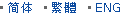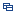| Recent Visit History | Join Audited Suppliers | Help | Region Guide
» » » » Nanjing Product List

106.

## Condenser Optical Glass Double Convex Lens[Nov 20, 2020]

Double Convex lens has a positive focal length, and with two outward surfaces. The two surfaces are with same radius of curvature, thereby minimizing spherical aberration. Some ...

107.

## Magnifier Double Convex Glass Lens[Nov 20, 2020]

Double Convex lens has a positive focal length, and with two outward surfaces. The two surfaces are with same radius of curvature, thereby minimizing spherical aberration. Some ...

108.

## Industrial Laser Optics Bi Concave Lens Optical Glass[Nov 20, 2020]

Bi concave lenses comprise of two inward equally curved surfaces. They are ideal for beam expansion, light projection and expanding the focal length of an optical system. Bi ...

109.

## Reflective Mirror Plano Concave Lens Optical Glass[Nov 20, 2020]

Plano-concave lenses are thicker at the edge than in the center and flat on another surface. Plano concave lens (PCV) has negative focal length. So the lenses are used to bend ...

110.

## Glass Lens-Bk7 Glass Lens-Fused Silica Glass Lens[Nov 20, 2020]

Bi concave lenses comprise of two inward equally curved surfaces. They are ideal for beam expansion, light projection and expanding the focal length of an optical system. Bi ...

111.

## Optical Glass Double Convex Lens Custom Made[Nov 20, 2020]

Double convex lenses have two outward curved surfaces, normally the two surfaces have same radius of curvature, with finely polished. Bi convex lenses have positive focal length, ...

112.

## Objective Lens Bi Convex Lens Optical Glass[Nov 20, 2020]

Double convex lenses have two outward curved surfaces, normally the two surfaces have same radius of curvature, with finely polished. Bi convex lenses have positive focal length, ...

113.

## Factory Optical Glass Bk7 Double Convex Lens[Nov 20, 2020]

Double Convex lens has a positive focal length, and with two outward surfaces. The two surfaces are with same radius of curvature, thereby minimizing spherical aberration. Some ...

114.

## Plano Concave Lens-Camera Lens-Glass Lens[Nov 20, 2020]

Plano-concave lenses are thicker at the edge than in the center and flat on another surface. Plano concave lens (PCV) has negative focal length. So the lenses are used to bend ...

115.

## Magnifying Lens-Magnifier Glass Lens Plano Convex Lens[Nov 20, 2020]

Spherical lenses include plano convex lens, plano concave lens, double convex lens, double concave lens, meniscus lens, and achromatic lens. Double convex lenses have two outward ...

116.

## Biconvex Lens Double Convex Lens Optical Glass[Nov 20, 2020]

Bi convex lenses have two outward curved surfaces, normally the two surfaces have same radius of curvature, with finely polished. Bi convex lenses have positive focal length, so ...

117.

## Manufacturer Bk7 Glass Bi Convex Lens[Nov 20, 2020]

Double convex lenses have two outward curved surfaces, normally the two surfaces have same radius of curvature, with finely polished. Bi convex lenses have positive focal length, ...

118.

## Manufacturer Optical Glass Bk7 Double Convex Lens[Nov 20, 2020]

Double Convex lens has a positive focal length, and with two outward surfaces. The two surfaces are with same radius of curvature, thereby minimizing spherical aberration. Some ...

119.

## Custom Made Plano Concave Lens Double Concave Lens Optical Glass[Nov 20, 2020]

Meniscus lenses are designed to minimize spherical aberration. When used in combination with another lens, meniscus lenses will shorten the focal length and increase the NA of the ...

120.

## Germanium Glass Bi Convex Lens[Nov 20, 2020]

Double convex lenses have two outward curved surfaces, normally the two surfaces have same radius of curvature, with finely polished. Bi convex lenses have positive focal length, ...

Hot Region Index: ABCDEFGHIJKLMNPQRSTUWXYZ